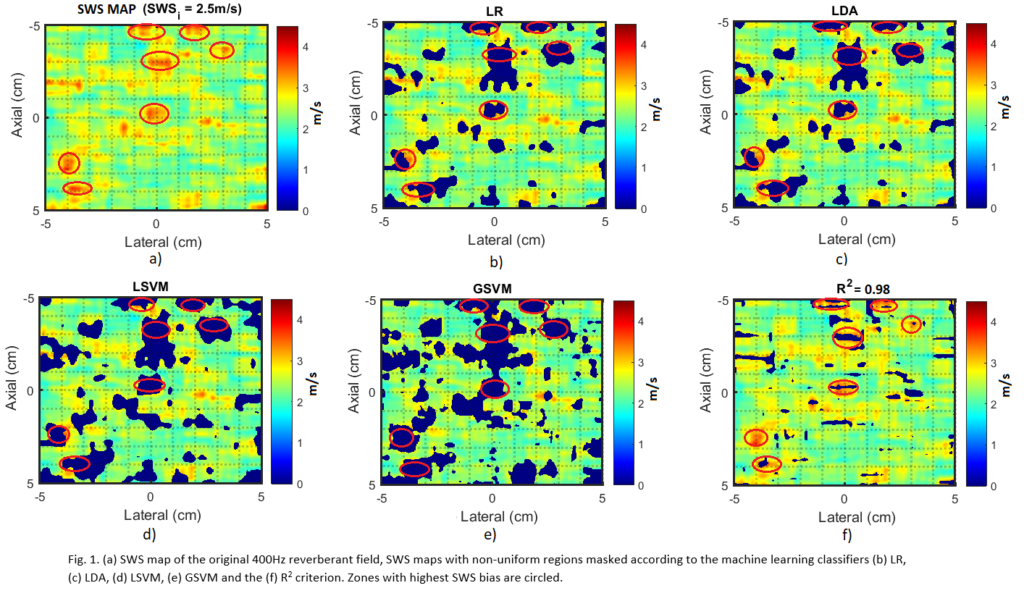# A New Statistical Approach for Detection of Uniform Reverberant Shear Wave Fields is accepted for IEEE IUS 2021 presentation

Fecha: 02 de septiembre del 2021Reverberant shear wave field elastography (R-SWE) evaluates tissue stiffness by creating a reverberant field in all directions of the medium which facilitates the estimation of the shear wave speed (SWS). Nevertheless, mathematical assumptions of the SWS estimation are valid if a uniform field is created. Current criterion uses the coefficient of determination (R^2) as a quality metric of reverberation. However, this metric is derived from a curve fitting of the autocorrelation of the field and does not directly evaluate its uniformity. Herein, we proposed the extraction of statistical estimators used in electromagnetic fields with their equivalency in acoustic waves to implement machine learning classifiers of uniform reverberant fields: Logistic Regression, LDA, Lineal SVM and Gaussian SVM. The models succeed on detecting regions with larger SWS bias decreasing the standard deviation of the SWS with accuracies of 0.75, 0.75, 0.74 and 0.80 for the LR, LDA, LSVM and GSVM, respectively.

Compartir vía:
Enviar a:

### Deja un comentario